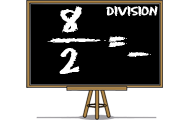# Mental calculationFiltering on knowledge field: Mental calculation

Math games that will teach you about mathematics, numbers and counting. Practice the basic number perception with an abacus, or practice the four rules of arithmetic. You will learn math in a joyful way by playing these games. Here we have collected all the games on the subject Math. The games suit preschool, elementary school and all the way up to high school. The games may be sorted by popularity, target group, release date or name.
0%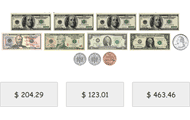## Count money

• Money
• Counting
• Mental calculation
Look at the notes and coins and add up the sum. Practicing basic knowledge in how to count money with increasing difficulty. This knowledge game uses all valid denominations of the Swedish krona, U.S.
0%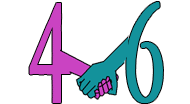## Number bonds

• Numbers
• Mental calculation
• Number bonds
Try to find the number that is a number bond to another number and adds up to the given sum. Practice part-part-whole relationships to the numbers 0-20 and facilitates the important mental calculation
0%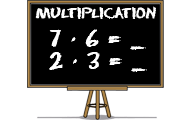## Multiplication

• Numbers
• Multiplication
• Mental calculation
Learn multiplication with this math game for students. Find the product of the factors in all times tables.
0%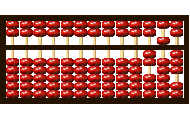## Abacus - Basics

• Logical thinking
• Mental calculation
• Abacus
Learn to count on an abacus online. In order to be able to count on an abacus you have to master the basics. In this game you train the values of the beads, the importance of the beam and the rods.
0%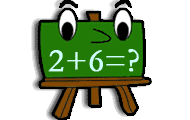• Numbers
• Mental calculation
Practice addition in math game with several levels of increasing difficulty. Add two numbers and find the correct sum.
0%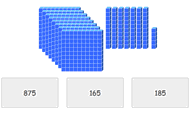## Place value with base ten blocks

• Counting
• Mental calculation
Look at the picture and count the hundreds. Then count the number of tens and after that the ones. Sum up and click on the correct number. Practice counting and improve your perception of numbers in t
0%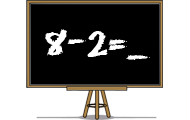## Subtraction

• Numbers
• Subtraction
• Mental calculation
Practice subtraction or minus in a skill training game. Find the difference between two numbers in different levels in this math game.
0%